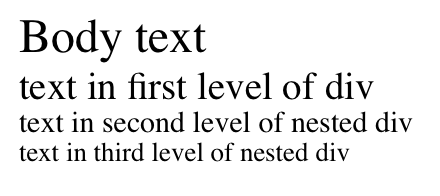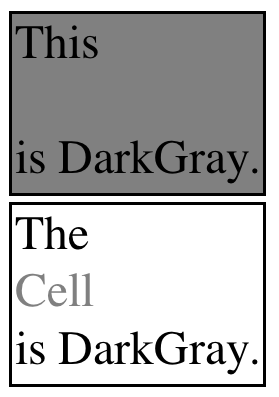# On the Analysis of Cascading Style Sheets

Pierre Genevès, CNRS
Nabil Layaïda, INRIA
Vincent Quint, INRIA

LIG Laboratory and INRIA Grenoble, France

International Conference on World Wide Web, April 2012

# Rough Timeline of Core Web Technologies

 1991: HTML 1994: HTML2 1996: CSS , Javascript 1997: HTML4 1998: CSS2 2000: XHTML1 2005: AJAX 2009: CSS3, HTML5 Initial foundations of the web: HTML, HTTP, URL First added feature: Cascading Style Sheets (CSS) Nowadays: HTML 5 + CSS3 + Javascript

# CSS Today• Increasingly important component of web user experience
• CSS3 offers new features: animations, transitions, transforms, text effects, opacity, color, gradients, shadows, background enhancements, borders, flexible box model, webfonts, columns, etc.
• A key component of the Open Web Platform
• Integration with HTML 5 paves the way for advanced (Flash-less) web graphic designs

# CSS in a Nutshell

CSS appears very simple at first sight:

• Everything is about setting pairs (property, value) for HTML elements
• HTML elements are identified using a selector:
• Easy to use
• Not rocket science
• Web designers' heaven
• See the tutorial by Bert Bos

# However...

CSS has intricate facets:

• cascade: properties of an element may be set by several rules from various origins (e.g. stylesheets)
• priorities: for a given property, rules have different priorities specified by vectors (specificity)
• inheritance: values may be propagated recursively
• powerful selector language (used for queries in JSON):
div p.contents ~ img
• pseudo-classes:
:nth-child(even)

# CSS in Practice

Web designers' heaven... but web developpers' hell

• Knowing which rules apply for a given element can be very hard
• CSS can be very difficult to debug

Today, CSS debugging technique = testing

• Testing consists in:
1. write a CSS
2. write a sample document
3. check the rendering visually
4. repeat the process until you get convinced/bored enough
• A variety of debugging tools (e.g. Firebug)

# Limits of current technology

• Very little basic research involved for CSS tools
• Empirical methods are the only debugging means available
• Testing does not prove general correctness:
• how many documents must be tested?
• which ones?
• how complex should they be?
• when to stop?

# A Notion of Correctness?

What would be "correctness" for CSS anyway?

• Usually a CSS is intended to be applied to a set of documents
• This whole set should be considered
• This set may be described by (optional) schema information (e.g. HTML DTD, or any XML Schema)

What would be an "incorrect" CSS?

• Style inconsistencies: unreadable text (undistinguishable color, font size too small...), broken box model, broken graphic charter: unexpected color for an element, font family is left unspecified...
• CSS code at risk (empty selectors, unexpected overlapping selectors...)

# Objectives and Challenges

Main objective: Find analysis methods that

• Analyze the CSS while capturing its intricate facets
• Consider the whole set of documents
• Automatically detect style inconsistencies and code at risk

Main difficulties

• Develop reasoning techniques supporting: selector navigation, inheritance, schemas
• Algorithmic/theoretical challenge: possibly infinitely many documents!

# Proposed Approach

 Describe CSS mechanisms and dependencies logically Formulate the existence of style inconsistencies Use a logical reasoner for solving satisfiability Possible outputs: Formula is unsatisfiable: CSS correctness proven Formula is satisfiable: style inconsistencies exist for some document(s), we generate the smallest counter-example SVG Architecture

# Which Logic?

Chosen logic:

• The $\mu$-calculus of trees proposed in [Geneves-PLDI'07]
• Initially developed for XPath static analysis
• Supports schemas (regular tree grammars)
• Satisfiability-testing is in EXPTIME ($2^{\mathcal{O}(n)}$ where $n=|\phi|$)
• Comes with an effective implementation (using symbolic techniques)

• Expressive enough for CSS3 selectors (we implemented a CSS3 compiler)
• Required an extension for CSS properties/values

# Zoom on Logical Formulas

XML trees are seen as labeled binary trees (wlog)

Programs $\alpha \in \{ 1, 2, \overline{1}, \overline{2}\}$ for navigation

A formula can be:

• true $\top$
• a label $\sigma$
• $\phi \wedge \phi$, $\neg\phi$
• an existential modality $\left<\alpha\right>\phi$
• a property $\left< prop\right>\top$, a property-value pair $\left< prop\right>\text{'v'}$
• a recursion $\mu X.\phi(X)$

# Translating CSS in the Logic

 Sample CSS rule: div p   {color: red;} Logical formula: $p \wedge \langle\text{color}\rangle \text{'red'} \wedge \mu X. \langle\overline{1}\rangle\text{div} \vee \langle\overline{2}\rangle X \vee \langle \overline{1}\rangle X$

Main principles:

• Formula holds at selected nodes
• $\mu$: navigation with unbounded number of steps
• Schemas can be translated as well
• Inheritance can be modeled with recursion

# Example with one Property

Sample CSS

 1 tr:nth-child(even) {background-color:LightGray;} 2 tr:nth-child(odd) {background-color:DarkGray;} 3 table td {font-size: 16px;} 4 td {font-size: 14px;} 5 div {color:DarkGray;} 6 div {font-size:smaller;}
• Given cascade, priorities, inheritance, can a property reach an unacceptable value for some document?
• Can font size go too small?
$\left.\begin{array}{l} \phi_\text{prop}(\text{<}\text{font-size}\text{>}\text{'8px'})\\ \phi_\text{CSS}(\text{<}\text{font-size}\text{>}) \\ \phi_\text{Schema} \end{array}\right\} \phi$
 $\rightarrow$ SVG solver
$\rightarrow$  satisfiable  $\rightarrow$
 Body text
text ...
text ...
text ...# Example with one Property (cont.)

Sample CSS

 1 tr:nth-child(even) {background-color:LightGray;} 2 tr:nth-child(odd) {background-color:DarkGray;} 3 table td {font-size: 16px;} 4 td {font-size: 14px;} 5 div {color:DarkGray;} 6 div {font-size:smaller;} 7 div div div {font-size:medium;}
• Can font size still go too small?
$\left.\begin{array}{l} \phi_\text{prop}(\text{<}\text{font-size}\text{>}\text{'8px'})\\ \phi_\text{CSS}(\text{<}\text{font-size}\text{>}) \\ \phi_\text{Schema} \end{array}\right\} \phi$
 $\rightarrow$ SVG solver
$\rightarrow$  unsatisfiable  $\rightarrow$
 No.There does not exist any document for which the font-size may go too small.

# Example with two Properties

Sample CSS

 1 tr:nth-child(even) {background-color:LightGray;} 2 tr:nth-child(odd) {background-color:DarkGray;} 3 table td {font-size: 16px;} 4 td {font-size: 14px;} 5 div {color:DarkGray;} 6 div {font-size:smaller;}
• Consider two properties: e.g. color and background-color
• Is there any chance that they interact in a bad way?
$\left.\begin{array}{l} \phi_\text{prop}(\text{<}\text{color}\text{>},\text{<}\text{bg-color}\text{>})\\ \phi_\text{CSS}(\text{<}\text{color}\text{>},\text{<}\text{bg-color}\text{>}) \\ \phi_\text{Schema} \end{array}\right\} \phi$
 $\rightarrow$ SVG solver
$\rightarrow$  sat.  $\rightarrow$
 ...
This
Cell
is DarkGray.
The
Cell
is DarkGray.
...# Detecting CSS Generic Issues

• Any complex case involving one, two, or more properties is treated similarly
• Style coverage: checks whether some elements are not covered by any selector (potentially breaking the intended design)
• Emptiness of selectors: every selector is tested against a given schema
• Equivalence of selectors/unreachable rules
• see the paper for more!
• Formulas can be automatically generated given a property
• Knowledge of the logic is not required

# Conclusion

• The first static analysis technique for CSS
• Allows one to check style properties for a whole set of documents (even infinite)
• Approach: reduction to logical satisfiability
• Theoretical computational complexity upper-bound: EXPTIME
• Implementation (online demo: http://wam.inrialpes.fr/websolver)
• Results embeddable in CSS editors
• Perspectives: support new CSS features e.g. CSS Variables, W3C Working Draft 10 April 2012

# Thank you!

Further information:

 Web site: http://wam.inrialpes.fr/websolver These slides: http://wam.inrialpes.fr/talks/2012/WWW12/slides.htm Email: pierre.geneves@inria.fr

# CSS Inheritance

• The particular property value "inherit" (not related to style) indicates how the property value must be computed
• Computed value $v$ is obtained for property $p$ at element $e$ iff:
1. $p$ is explicitly set to $v$ at $e$ (with some custom selector)
2. $p$ is not explicitly set to $v$, it is not set to inherit, but $p$ initial value is $v$
3. $p$ is set to inherit at $e$, $e$ is the root, $p$ initial value is $v$
4. $p$ is set to inherit at $e$, $e$ $\neq$ root, $v$ is obtained for $e$'s parent (by recursion)
• In logic:
•  cases1-3(v,p) $\stackrel{\text{def}}{=} \left\{\begin{array}{ll} \langle p\rangle \text{'}v\text{'} \vee \neg \langle p\rangle \text{'}v\text{'} \wedge \neg \langle p\rangle \text{'inherit'} \wedge \text{initialvalue}(p,v) \\ ~~\vee \langle p\rangle \text{'inherit'} \wedge \neg \langle\overline{1}\rangle \top \wedge \neg \langle\overline{2}\rangle \top \wedge \text{initialvalue}(p,v)\end{array}\right.$ $\text{initialvalue}(p,v) \stackrel{\text{def}}{=}p$ has initial value $v$ (cf. CSS recommendation) obtained(v,p) $\stackrel{\text{def}}{=}$ cases1-3(v,p) $\vee \langle p\rangle \text{'inherit'} \wedge$ $\mu X. \langle \overline{1}\rangle\big($ cases1-3(v,p) $\vee \langle p\rangle \text{'inherit'} \wedge X \big) \vee \langle\overline{2}\rangle X$

# Compiling Main CSS Combinators

$\begin{array}{lll} \textbf{Semantics} & \textbf{CSS} & \textbf{Tree Logic} \\ \hline \text{Any element} & \text{*} & \top \\ \text{Any 'p' element} & \text{p} & \text{p} \\ \text{Any child of some p element} & \text{p > *} & \mu X. \langle\overline{1}\rangle p \vee \langle\overline{2}\rangle X \\ \text{Any descendant 'b' of some 'a' element} & \text{a b} & b \wedge \mu X. \langle \overline{1}\rangle (a \vee X) \vee \langle \overline{2}\rangle X \\ \text{Any element with class 'foo' } & \text{.foo} & \langle \text{class}\rangle '\text{foo}' \\ \text{Any element with attribute 'title' } & \text{*[title]} & \langle \text{title}\rangle \top\\ \text{Any 'p' element with an 'a' child} & \text{Not possible} \qquad & p \wedge \langle 1\rangle \mu X. a \vee \langle 2\rangle X\\ \text{Any adjacent next sibling of a 'p' element} & \text{p + *} & \langle \overline{2}\rangle p \\ \text{Any next sibling 'pre' of a 'h1' element} \qquad & \text{h1 ~ pre} & \text{pre} \wedge \mu X. \langle \overline{2}\rangle \text{h1} \vee \langle \overline{2}\rangle X \\ \text{Any 'e' whose 'foo' attribute value is 'bar'} \qquad & \text{e[foo="bar"]} & e \wedge \langle \text{foo}\rangle'\text{bar}' \end{array}$